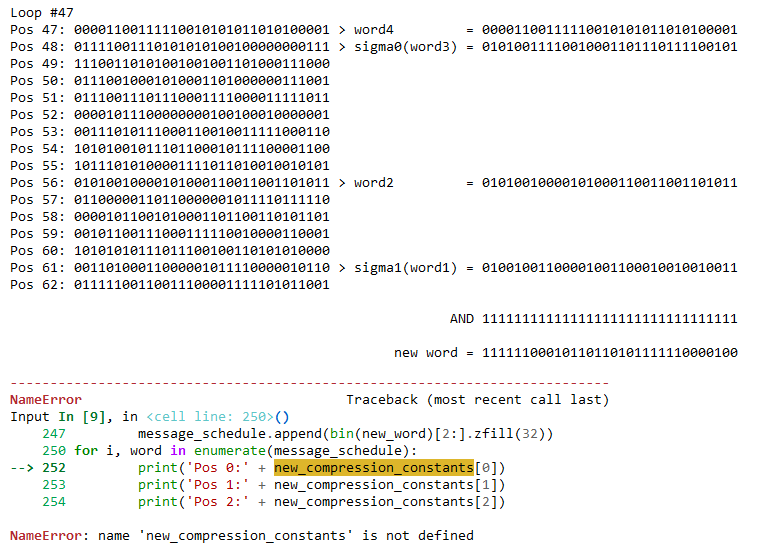Instantly share code, notes, and snippets.

# nickyreinert/01 init.ipynb

Last active February 5, 2023 20:59
Step-by-Step implementation of the SHA-256 algorithm in Python
This file contains bidirectional Unicode text that may be interpreted or compiled differently than what appears below. To review, open the file in an editor that reveals hidden Unicode characters. Learn more about bidirectional Unicode characters
 message = 'hello_world' from math import floor from IPython.display import clear_output import time def rotate(value, rotations, width=32): # thx to https://stackoverflow.com/a/59005609/2360229 if int(rotations) != abs(int(rotations)): rotations = width + int(rotations) return (int(value) << (width - (rotations%width)) | (int(value) >> (rotations % width))) & ((1 << width) - 1) def sigma0(word): part1 = bin(rotate(int(word, 2), 7, 32)) part2 = bin(rotate(int(word, 2), 18, 32)) part3 = bin(int(word, 2) >> 3) return bin(int(part1, 2) ^ int(part2, 2) ^ int(part3, 2))[2:].zfill(32) def sigma1(word): part1 = bin(rotate(int(word, 2), 17, 32)) part2 = bin(rotate(int(word, 2), 19, 32)) part3 = bin(int(word, 2) >> 10) return bin(int(part1, 2) ^ int(part2, 2) ^ int(part3, 2))[2:].zfill(32) def upper_sigma0(word): part1 = bin(rotate(int(word, 2), 2, 32)) part2 = bin(rotate(int(word, 2), 13, 32)) part3 = bin(rotate(int(word, 2), 22, 32)) return bin(int(part1, 2) ^ int(part2, 2) ^ int(part3, 2))[2:].zfill(32) def upper_sigma1(word): part1 = bin(rotate(int(word, 2), 6, 32)) part2 = bin(rotate(int(word, 2), 11, 32)) part3 = bin(rotate(int(word, 2), 25, 32)) return bin(int(part1, 2) ^ int(part2, 2) ^ int(part3, 2))[2:].zfill(32) def choose(word1, word2, word3): bin_word1 = (int(word1, 2)) bin_word2 = (int(word2, 2)) bin_word3 = (int(word3, 2)) return bin((bin_word1 & bin_word2) ^ (~bin_word1 & bin_word3))[2:].zfill(32) def majority(word1, word2, word3): bin_word1 = (int(word1, 2)) bin_word2 = (int(word2, 2)) bin_word3 = (int(word3, 2)) return bin((bin_word1 & bin_word2) ^ (bin_word1 & bin_word3) ^ (bin_word2 & bin_word3))[2:].zfill(32) first_64_prime_numbers = [2, 3, 5, 7, 11, 13, 17, 19, 23, 29, 31, 37, 41, 43, 47, 53, 59, 61, 67, 71, 73, 79, 83, 89, 97, 101, 103, 107, 109, 113, 127, 131, 137, 139, 149, 151, 157, 163, 167, 173, 179, 181, 191, 193, 197, 199, 211, 223, 227, 229, 233, 239, 241, 251, 257, 263, 269, 271, 277, 281, 283, 293, 307, 311] result_constants = [] for prime_number in first_64_prime_numbers: # get cube root cube_root = prime_number ** (1./3.) # get fractional part frac_part = cube_root - floor(cube_root) # multiply with 2^32 (or shift left by 32 bits) product = frac_part * (2**32) floored_product = floor(product) # floor, ready: result_constants.append(bin(floored_product)[2:].zfill(32)) first_8_prime_numbers = [2, 3, 5, 7, 11, 13, 17, 19] compression_constants = [] for prime_number in first_8_prime_numbers: # get square root square_root = prime_number ** (1./2.) # get fractional part frac_part = square_root - floor(square_root) # multiply with 2^32 (or shift left by 32 bits) product = frac_part * (2**32) floored_product = floor(product) compression_constants.append(bin(floored_product)[2:].zfill(32))
Sorry, we cannot display this file.
Sorry, this file is invalid so it cannot be displayed.
Sorry, we cannot display this file.
Sorry, this file is invalid so it cannot be displayed.
Sorry, we cannot display this file.
Sorry, this file is invalid so it cannot be displayed.
Sorry, we cannot display this file.
Sorry, this file is invalid so it cannot be displayed.
Sorry, we cannot display this file.
Sorry, this file is invalid so it cannot be displayed.
Sorry, we cannot display this file.
Sorry, this file is invalid so it cannot be displayed.

### Tardis4Future commented Aug 5, 2022 • edited

Hello, i got message that this notebook is invalid. I can not open it in jupyter.